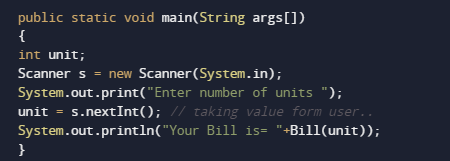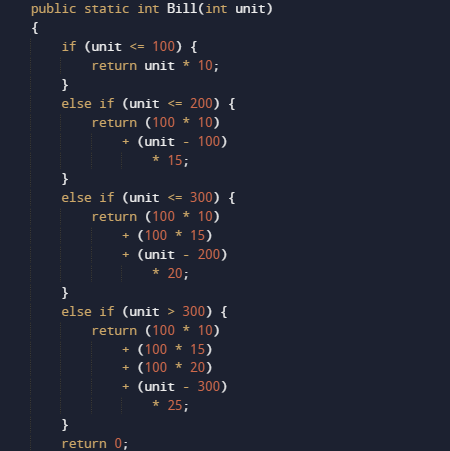# Create Electric Bill Calculator Program In java

In this tutorial you will learn about the Create Electric Bill Calculator Program In java and its application with practical example.

In this tutorial, we will learn to Create a program of Electric Bill Calculator Program In java  using Java programming.

## Prerequisites

Before starting with this tutorial we assume that you are best aware of the following Java programming topics:

• Java Operators.
• Basic Input and Output function in Java.
• Class and Object in Java.
• Basic Java programming.
• If-else statements in Java.
• nested if-else statements.
• Loop’s in Java.

## How to calculate Electricity bill.

Here in this tutorial you will learn to calculate Electricity bill by simply writing  Java program . Create Electric Bill Calculator Program In java

Before, writing a program.

first we know about the tariff rates of per unit according to MPEB .Here we are taking some random charges  user can alter according to their Electric Broad.

• 1 to 100 units –rs 10/unit.
• 100 to 200 units –rs 15/unit.
• 200 to 300 units – rs 20/unit.
• above 300 units – rs 25/unit.

If consumer use electricity between “0-100” unit then he/she have to pay 10 rs per unit, if we consume electricity between 100-200 unit then for the first 100 he/she have to pay 10 per unit (i.e. 100*10), and for remaining pay 15 per unit. Similarly, if consume >200 units then he/she have to pay 10 per unit for the first 100 units 15 per unit for the next 100 units, and 20 per unit for the remaining units. and so on..

## Java program to calculate Electricity bill

In this program we will to create a java program to generate electric Bill . We would first declared and initialized the required variables. Next, we would prompt user to input the units. Later we will Calculate Bill.

## OutputIn the above program, we have first declared and initialized a set variables required in the program.

• unit= it will hold entered units.After that we pass the given unit by user to a user define function “Bill(unit)”where we calculate Bill according to provided units using nested if-else statement and after calculate bill reruns the bill to main function.here we will print the charge payable by user for consuming that number of unitsIn this tutorial we have learn about the Create Electric Bill Calculator Program In java and its application with practical example. I hope you will like this tutorial.